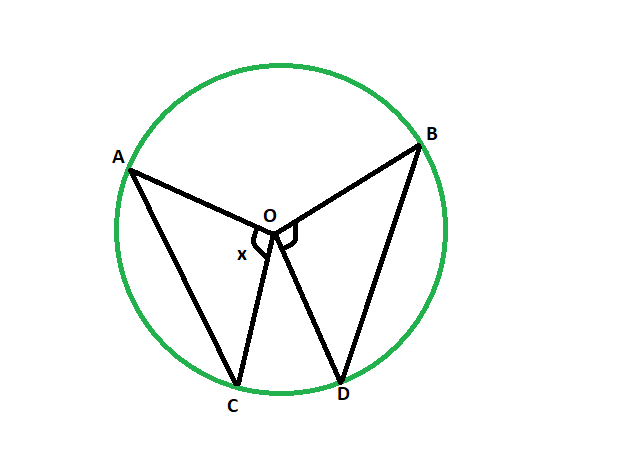# Angle subtended by the chord when the angle subtended by another chord of same length is given

Given a circle having two chords of equal length. The angle subtended by one of the chords to the centre is given. The task here is to find the measure of the angle subtended by another chord at the centre.

Examples:

```Input: 48
Output: 48 degrees

Input: 82
Output: 82 degrees

```Approach:
Let AC & BD are the two equal chords of the circle having center at O.let the angle subtended by the chord AC be x degrees.
now,
in triangle AOC & BOD,
AO = OB(radii of same circle)
AB = CD(equal chords)
OC = OD(radii of same circle)
so, the triangles AOC & BOD are congruent to each other
so, angle AOC = angle BOD

Equal chords of a circle subtend equal angles at the centre.

Below is the implementation of the above approach:

## C++

 `// C++ program to find the angle ` `// subtended at the center by the chord ` `// when the angle subtended at center ` `// by another chord of equal length is given ` ` `  `#include ` `using` `namespace` `std; ` ` `  `void` `angleequichord(``int` `z) ` `{ ` `    ``cout << ``"The angle subtended at the center is "` `         ``<< z << ``" degrees"` `<< endl; ` `} ` ` `  `// Driver code ` `int` `main() ` `{ ` `    ``int` `z = 48; ` `    ``angleequichord(z); ` `    ``return` `0; ` `} `

## Java

 `// Java program to find the angle ` `// subtended at the center by the chord ` `// when the angle subtended at center ` `// by another chord of equal length is given ` ` `  `import` `java.io.*; ` ` `  `class` `GFG  ` `{ ` `     `  `static` `void` `angleequichord(``int` `z) ` `{ ` `    ``System.out.println ( ``"The angle subtended at the center is "``+ ` `        ``z + ``" degrees"``); ` `} ` ` `  `// Driver code ` `public` `static` `void` `main (String[] args)  ` `{ ` ` `  `    ``int` `z = ``48``; ` `    ``angleequichord(z); ` `} ` `} ` ` `  `// This code is contributed by ajit. `

## Python3

 `# Python3 program to find the angle  ` `# subtended at the center by the chord  ` `# when the angle subtended at center  ` `# by another chord of equal length is given  ` ` `  `def` `angleequichord(z) :  ` ` `  `    ``print``(``"The angle subtended at"``,  ` `          ``"the center is"``, z ,``"degrees"` `); ` ` `  `# Driver code  ` `if` `__name__ ``=``=` `"__main__"` `:  ` `     `  `    ``z ``=` `48``;  ` `    ``angleequichord(z);  ` `     `  `# This code is contributed by AnkitRai01 `

## C#

 `// C# program to find the angle ` `// subtended at the center by the chord ` `// when the angle subtended at center ` `// by another chord of equal length is given ` `using` `System; ` `     `  `class` `GFG  ` `{ ` `     `  `static` `void` `angleequichord(``int` `z) ` `{ ` `    ``Console.WriteLine( ``"The angle subtended at the center is "``+ ` `        ``z + ``" degrees"``); ` `} ` ` `  `// Driver code ` `public` `static` `void` `Main (String[] args)  ` `{ ` ` `  `    ``int` `z = 48; ` `    ``angleequichord(z); ` `} ` `} ` ` `  `/* This code contributed by PrinciRaj1992 */`

Output:

```The angle subtended at the center is 48 degrees
```

Attention reader! Don’t stop learning now. Get hold of all the important DSA concepts with the DSA Self Paced Course at a student-friendly price and become industry ready.

My Personal Notes arrow_drop_upCheck out this Author's contributed articles.

If you like GeeksforGeeks and would like to contribute, you can also write an article using contribute.geeksforgeeks.org or mail your article to contribute@geeksforgeeks.org. See your article appearing on the GeeksforGeeks main page and help other Geeks.

Please Improve this article if you find anything incorrect by clicking on the "Improve Article" button below.

Article Tags :
Practice Tags :

Be the First to upvote.

Please write to us at contribute@geeksforgeeks.org to report any issue with the above content.